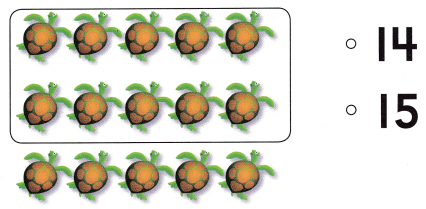# Texas Go Math Kindergarten Lesson 7.5 Answer Key Model, Count and Write 15

Refer to our Texas Go Math Kindergarten Answer Key Pdf to score good marks in the exams. Test yourself by practicing the problems from Texas Go Math Kindergarten Lesson 7.5 Answer Key Model, Count and Write 15.

## Texas Go Math Kindergarten Lesson 7.5 Answer Key Model, Count and Write 15

Explore

DIRECTIONS: Use counters to show the number 15. Draw the counters. Trace and write the number. Trace the word.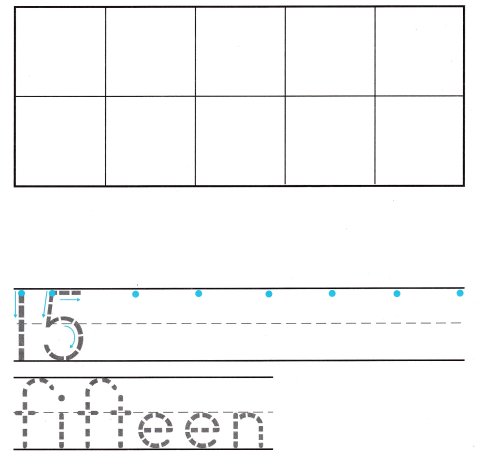Explanation:
Number of counters = 15 or Fifteen.

Share and Show

DIRECTIONS: 1. Count and tell how many. Write the number. 2. Use counters to show the number 15. Draw the counters. 3. Look at the counters you drew. How many are in the ten frame? Write the number. How many more? Write the number.

Question 1.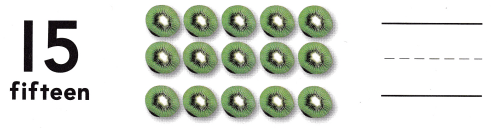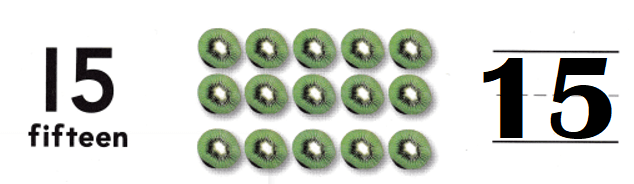Explanation:
Number of kiwi fruits = 15 or Fifteen.

Question 2.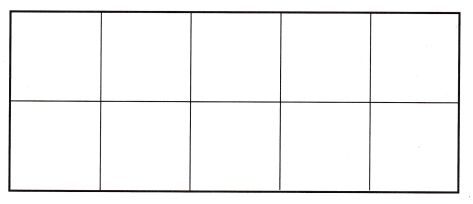Explanation:
Number of counters = 15 or Fifteen.

Question 3.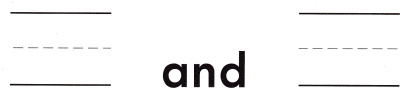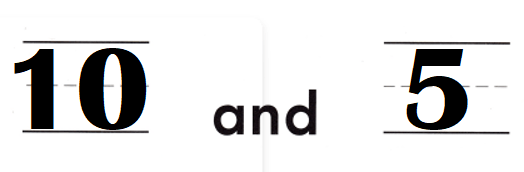Explanation:
Number of counters in frame = 10.
Number of counters out of frame = 5.
Total number of counters = Number of counters in frame + Number of counters out of frame
= 10 + 5
= 15.

DIRECTIONS: 4-5. Count and tell how many. Write the number. 6. Look at the ten circles and some more in Exercise 5. Write how many blue circles. Write how many red circles. Write how many in all.

Question 4.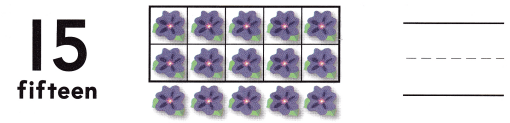Explanation:
Number of purple flowers = 15 or Fifteen.

Question 5.Explanation:
Number of blue counters = 10.
Number of red counters = 5.
Total number of counters = Number of blue counters + Number of red counters
= 10 + 5
= 15 or Fifteen.

Question 6.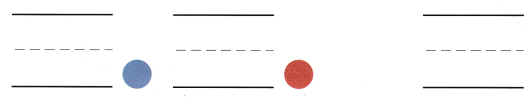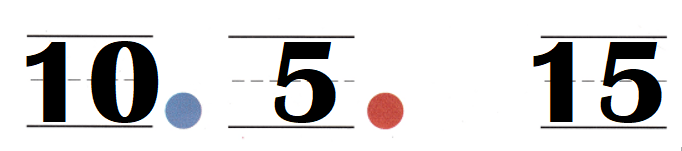Explanation:
Number of blue counters = 10.
Number of red counters = 5.
Total number of counters = Number of blue counters + Number of red counters
= 10 + 5
= 15 or Fifteen.

HOME ACTIVITY • Have your child use two different kinds of objects to show all the ways he or she can make 15, such as 8 pieces of macaroni and 7 pieces of bowtie pasta.
Total number of objects = 15 or Fifteen.

Explanation:
Number of books = 8.
Number of pencils = 7.
Total number of objects = Number of books  + Number of pencils
= 8 + 7
= 15 or Fifteen.

Problem Solving
Question 7.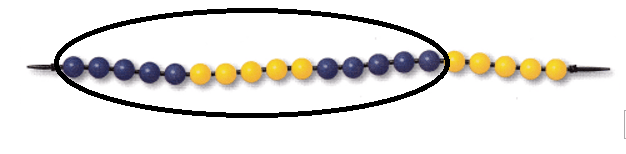Explanation:
Number of blue beads = 10.
Number of yellow beads = 5.
= 10 + 5
= 15 or Fifteen.

Question 8.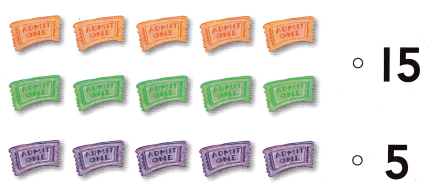Explanation:
Number of chocolates = 15 or Fifteen.

### Texas Go Math Kindergarten Lesson 7.5 Homework and Practice Answer Key

DIRECTIONS: 1. Draw counters to show 15. Write the number. 2. Draw counters to show 14. Write the number.

Question 1.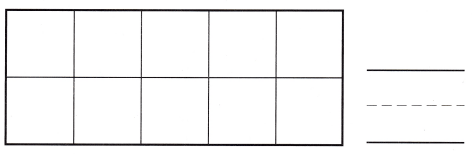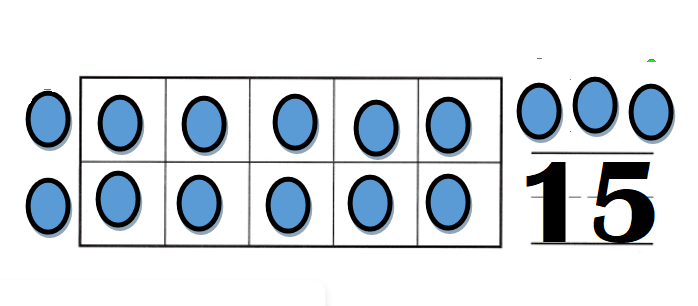Explanation:
Number of counters = 15 or Fifteen.

Question 2.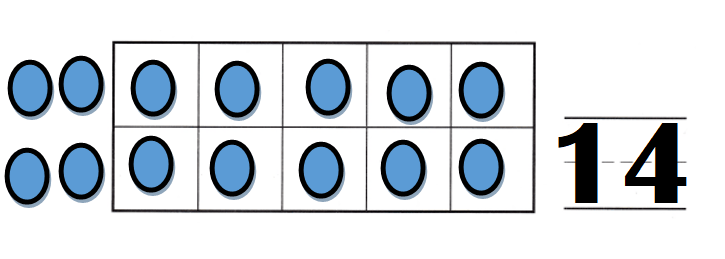Explanation:
Number of counters = 14 or Fourteen.

DIRECTIONS: Choose the correct answer. 3. Count the ants. How many are there? 4. Count the butterflies. How many are there? 5. Count the turtles. How many are there?

Lesson Check
Question 3.Explanation:
Number of ants = 13 or Thirteen.

Question 4.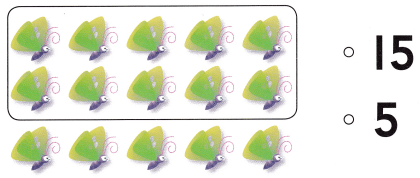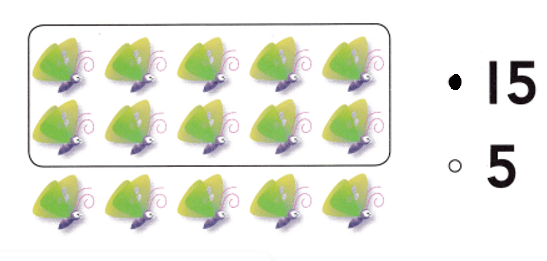Explanation:
Number of butterflies = 15 or Fifteen.

Question 5.